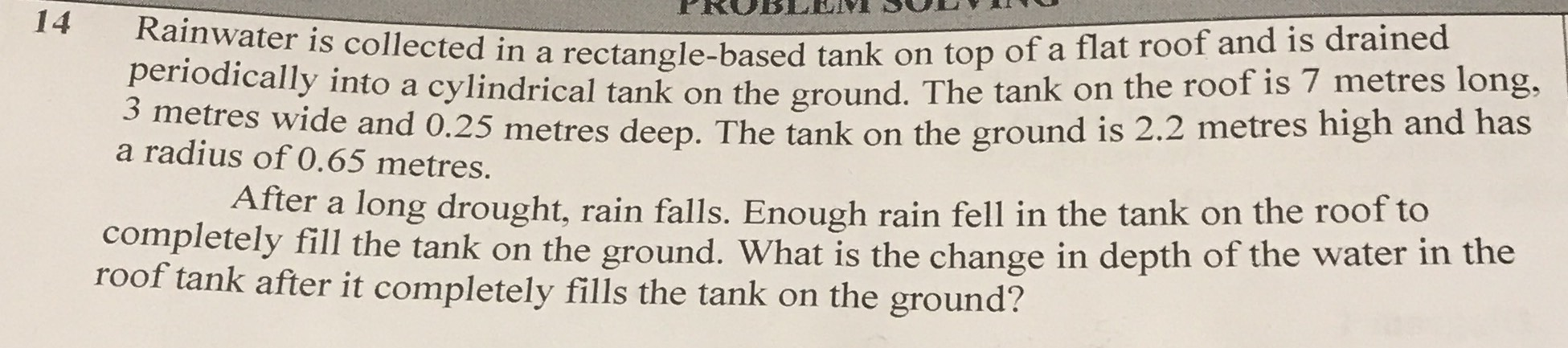### Still have math questions?

Arithmetic
Question14 Rainwater is collected in a rectangle-based tank on top of a flat roof and is drained periodically into a cylindrical tank on the ground. The tank on the roof is $$7$$ metres long, $$3$$ metres wide and $$0.25$$ metres deep. The tank on the ground is $$2.2$$ metres high and has a radius of $$0.65$$ metres. After a long drought, rain falls. Enough rain fell in the tank on the roof to completely fill the tank on the ground. What is the change in depth of the water in the roof tank after it completely fills the tank on the ground?

$$3.14 \cdot 0.65 ^ { 2 } \cdot 2.2 \cdot \frac { 1 } { 3 \cdot 7 } = 0.13898$$